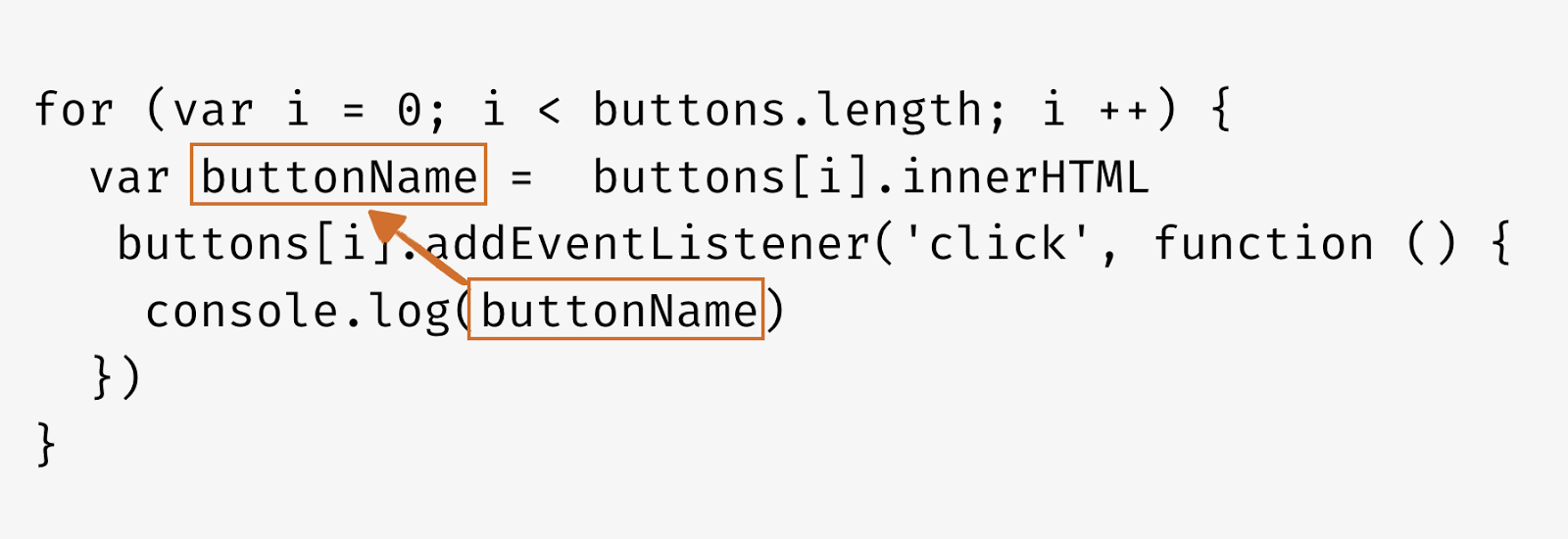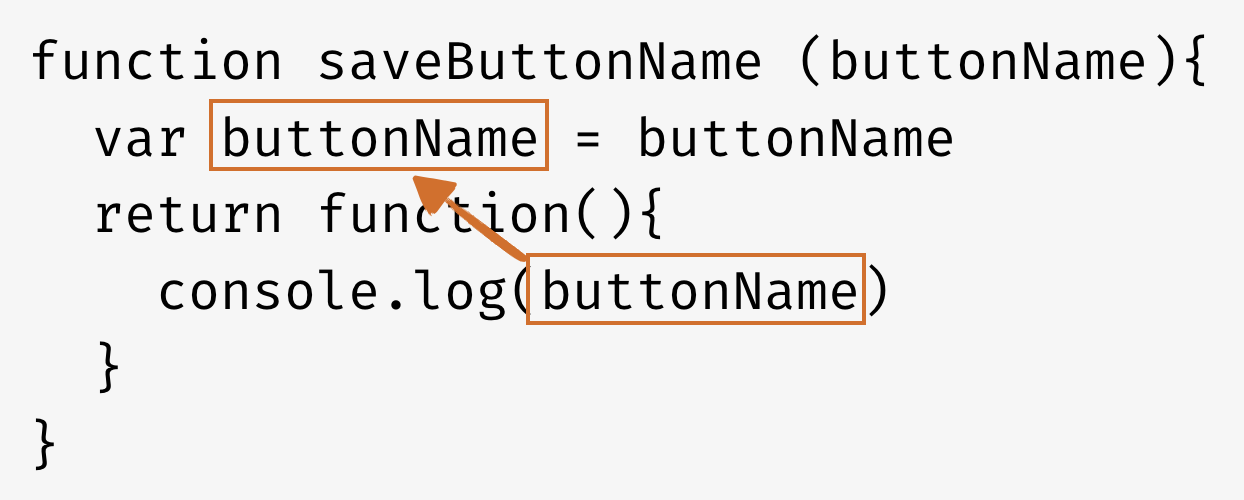# 深入淺出瞭解 JavaScript 閉包（closure）

## 不使用閉包（closure）的情況

``````// 狗的計數程式
var count = 0

function countDogs () {
count += 1
console.log(count + ' dog(s)')
}

countDogs()    // 1 dog(s)
countDogs()    // 2 dog(s)
countDogs()    // 3 dog(s)
``````

``````// 狗的計數函式
var count = 0

function countDogs () {
count += 1
console.log(count + ' dog(s)')
}

// 中間是其他程式碼...

// 貓的計數函式
var count = 0

function countCats () {
count += 1
console.log(count + ' cat(s)')
}

countCats()    // 1 cat(s)
countCats()    // 2 cat(s)
countCats()    // 3 cat(s)

``````

``````var count = 0

function countDogs () {
count += 1
console.log(count + ' dog(s)')
}

// 中間是其他程式碼...

var count = 0

function countCats () {
count += 1
console.log(count + ' cat(s)')
}

countCats()    // 1 cat(s)
countCats()    // 2 cat(s)
countCats()    // 3 cat(s)

countDogs()    // 4 dog(s)，我希望是 1 dog(s)
countDogs()    // 5 dog(s)，我希望是 2 dog(s)

countCats()    // 6 cat(s)，我希望是 4 cat(s)
``````

## 透過閉包讓 function 能夠有 private 變數

``````function dogHouse () {
var count = 0
function countDogs () {
count += 1
console.log(count + ' dogs')
}
return countDogs
}

const countDogs = dogHouse()
countDogs()    // "1 dogs"
countDogs()    // "2 dogs"
countDogs()    // "3 dogs"
````````````function dogHouse () {
var count = 0
function countDogs () {
count += 1
console.log(count + ' dogs')
}
return countDogs
}

function catHouse () {
var count = 0
function countCats () {
count += 1
console.log(count + ' cats')
}
return countCats
}

const countDogs = dogHouse()
const countCats = catHouse()

countDogs()    // "1 dogs"
countDogs()    // "2 dogs"
countDogs()    // "3 dogs"

countCats()    // "1 cats"
countCats()    // "2 cats"

countDogs()    // "4 dogs"
``````

## 進一步瞭解和使用閉包

``````function dogHouse () {
var count = 0
function countDogs () {
count += 1
console.log(count + ' dogs')
}
return countDogs
}

// 雖然都是使用 dogHouse ，但是各是不同的執行環境
// 因此彼此的變數不會互相干擾

var countGolden = dogHouse()
var countPug = dogHouse()
var countPuppy = dogHouse()

countGolden()     // 1 dogs
countGolden()     // 2 dogs

countPug()        // 1 dogs
countPuppy()      // 1 dogs

countGolden()     // 3 dogs
countPug()        // 2 dogs

``````

## 將參數代入閉包中

``````// 透過函式的參數將值代入閉包中
function dogHouse (name) {
var count = 0
function countDogs () {
count += 1
console.log(count + ' ' + name)
}
return countDogs
}

// 同樣是使用 dogHouse 但是使用不同的參數
var countGolden = dogHouse('Golden')
var countPug = dogHouse('Pug')
var countPuppy = dogHouse('Puppy')

// 結果更清楚了
countGolden()     // 1 Golden
countGolden()     // 2 Golden

countPug()        // 1 Pug
countPuppy()      // 1 Puppy

countGolden()     // 3 Golden
countPug()        // 2 Pug
``````

## 進一步簡化程式

### 直接 return function``````function dogHouse () {
var count = 0
// 把原本 countDogs 函式改成匿名函式直接放進來
return function () {
count += 1
console.log(count + ' dogs')
}
}

function catHouse () {
var count = 0
// 把原本 countCats 函式改成匿名函式直接放進來
return function () {
count += 1
console.log(count + ' cats')
}
}
``````

``````function createCounter (name) {
var count = 0
return function () {
count++
console.log(count + ' ' + name)
}
}

const dogCounter = createCounter('dog')
const catCounter = createCounter('cat')
const birdCounter = createCounter('bird')

dogCounter()     // 1 dog
dogCounter()     // 2 dog
catCounter()     // 1 cat
catCounter()     // 2 cat
birdCounter()    // 1 brid
dogCounter()     // 3 dog
catCounter()     // 3 cat
``````

## 閉包的實際應用

``````<button id="first">First</button>
<button id="second">Second</button>
<button id="third">Third</button>
``````

``````var buttons = document.getElementsByTagName('button')

for (var i = 0; i < buttons.length; i ++) {
// buttonName 暴露於 global environment
var buttonName =  buttons[i].innerHTML
console.log(buttonName)
})
}
````````````// 建立一個閉包把資料存在這個 function 當中
function saveButtonName (buttonName){
// buttonName 被儲存在閉包當中
var buttonName = buttonName
return function(){
console.log(buttonName)
}
}

var buttons = document.getElementsByTagName('button')
for (var i = 0; i < buttons.length; i ++) {
var buttonName =  buttons[i].innerHTML
}
``````### 使用 let

``````// 使用 ES6 寫法
for (var i = 0; i < buttons.length; i ++) {
let buttonName =  buttons[i].innerHTML